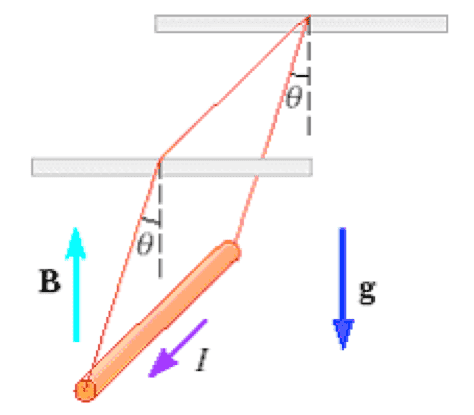# Magnetic Field Problem

• Heath90
In summary, Heath had difficulty with a magnetic field problem and was seeking help from others. If anyone could help him out, that would be great.f

#### Heath90

HI my name is Heath, I have an exam over Magnetic fields tomorrow. I was having problems on this one specific problem. If anybody could give me suggestions on how to solve that would be great.

A 2 m long metal rod having a mass per unit length λ = 65 g/m carries a current I = 1 A. The rod hangs from two vertical wires in a uniform vertical magnetic field as shown. The wires make an angle θ = 10 degrees with the vertical when in equilibrium. Determine the magnitude of the magnetic field. (Answer in Teslas.)

-I am unsure of what to do here I used the right hand rule and found the direction of Fb force vector.
- Do i need to do summation of forces to find B? Or is there a quicker way by simply manipulating the equation FB=ILxB?

I appreciate any input, thanks guys

I don't see the picture mentioned in the problem...I presume that there is information there that tells you the force...you know, they mention that the vertical wires are 10degrees when in equilibrium...is that what the picture shows? If not, they are showing you the position when the magnetic field is active and so a new position thanks to the force or something...there is only so much I can speculate.Heres the diagram attached to this file

I know which way Fb is pointing I just don't know how to use it to solve for B the magnetic field

did you calculate the weight of the rod? they gave you the mass per unit length and the length...that along with gravity and the angles and a free body diagram should tell you exactly the magnitude of the magnetic force from where you should be able to back calculate the magnetic field.

Yea I did, I just don't understand what the angles have to do with anything, G and B are vertical components, and the force would be going perpendicular to the rod. I am just confused on how the angles come into play.

the angles come into play because gravity is always point down and depending on the angle a component of the weight of the rod is going to be along the length of the wires used to hang the rod and being carried by the ceiling...the other component is the one that the magnetic force is balancing.

sorry...correction...the vertical component of the weight is going to be carried by the ceiling...the horizontal component will be balanced by the magnetic field.

Okay that makes sense, what about the angles on FB? I set up my axis so Fb is along the x axis, and G and B are on the Y axis.